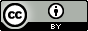# C++ 的关键字 mutable

## mutable 的特性

mutable 是个很有意思的属性。mutable 声明的类成员可以在 const 方法中被修改；const 实例中的 mutable 成员不受 const 的限制。

``````class C
{
public:
C():x(1),y(2){}

void func() const // 注意这里的 const
{
x = 3; // error: read-only variable is not assignable
y = 4; // ok
}

int x;
mutable int y;
};

int main()
{
const C c; // 这里也有 const

c.x = 5; // error: read-only variable is not assignable
c.y = 6; // ok

c.func();

return 0;
}

``````

## 适用场景

``````class C
{
public:
C():_size(0),_capacity(0){}

int size() const // const 允许 status() 对其访问
{
return _size;
}

int capacity() const // const 允许 status() 对其访问
{
return _capacity;
}

private:
int _size;
int _capacity;
};

void status(const C &c) // const 保证 status 不会改变 c 的内容
{
printf("size=%d, capacity=%d\n", c.size(), c.capacity());
}
``````

### 适用场景一

``````class C
{
//...

int capacity() const
{
if (_capacity == 0)
{
_capacity = ...; // 计算容量值，修改 _capacity
}
return _capacity;
}

private:
int _size;
mutable int _capacity; // 因为需要在 const 方法中被修改，所以必须有 mutable 属性
};

``````

### 适用场景二

``````class C
{
public:
C():_size(0),_capacity(0){}

//...

int size() const
{
int s = 0;

_lock.lock(); // lock() 会修改 _lock
s = _size;
_lock.unlock();

return s;
}

private:
int _size;
mutable Lock _lock; // 因为需要在 const 方法中被修改，所以必须有 mutable 属性
};
``````

## C++11 中的 mutable

``````{
int x = 0;
auto l1 = [=]() mutable { x = 1; };
auto l2 = [=]()         { x = 2; }; // error: cannot assign to a variable captured by copy in a non-mutable lambda

// ...
}
``````

## 总结

• mutable 声明的类成员可以在 const 方法中被修改。
• const 实例中的 mutable 成员不受 const 的限制。
• 适用场景一：当第一次请求时需要计算并缓存，以后只需访问缓存中的值。
• 适用场景二：const 方法需要修改类成员，而这种修改不需要、也不应该对调用者可见。
• 在 C++11 中，mutable 还会用在 Lambda 表达式中，用来允许执行体修改捕获的参数。Except where otherwise noted, content on this site is licensed under a Creative Commons Attribution 4.0 International license .